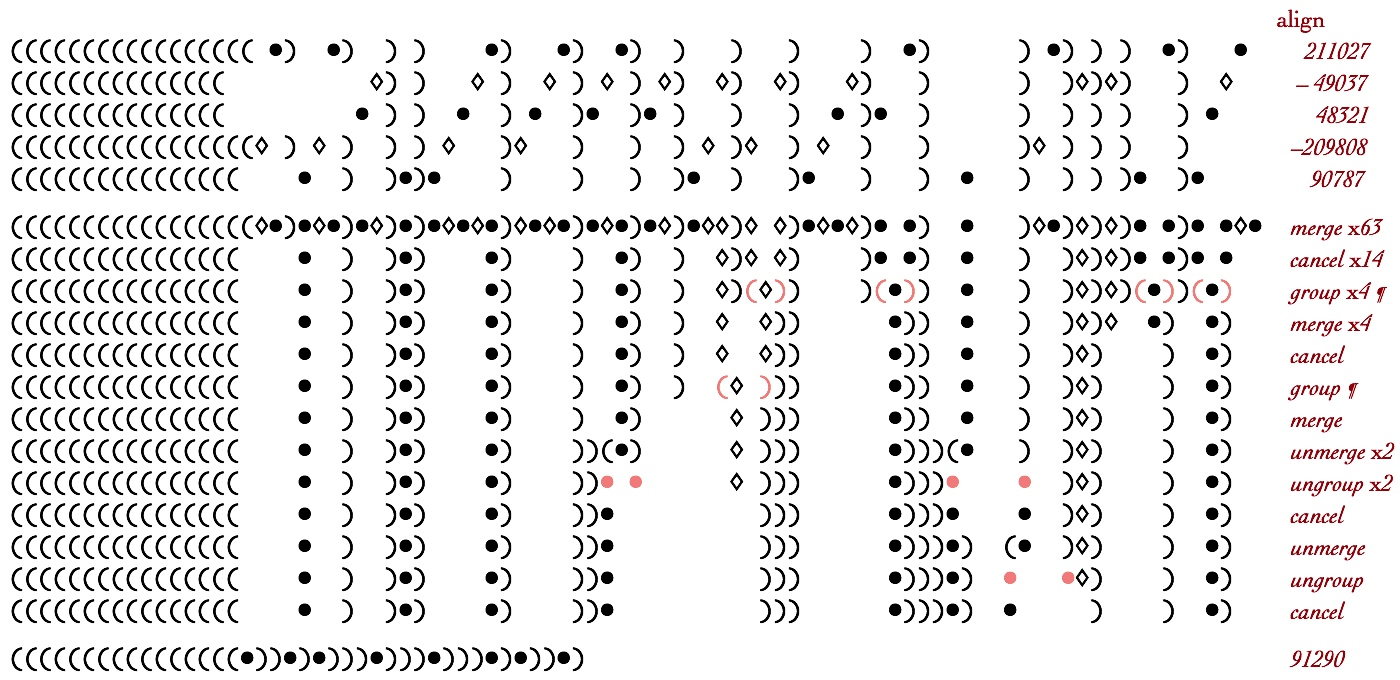# Parens Notation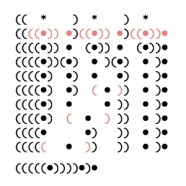Parens notation is a textual representation of containers that uses parentheses as delimiting tokens to stand in place of surrounding containers. In the Image, configurations are illustrated on each line, while the temporal evolution of applying transformations to parens configurations is shown as the stepwise progression of changed configurations going down the page. A parens demonstration shows an animation, with time spread in the vertical direction and with some parallelism suppressed. Iconic forms can be illustrated using linear text, using planar enclosures, or using solid objects. For example, the binary number 6 expressed below in one, two, and three dimensions. One-dimensional parens configurations represent the application of rules by changing the sequence of symbols in the textual expression. Two-dimensional container configurations express change by animation. Three-dimensional block configurations are intended to express change as physical activities. Thus iconic transformation rules can be expressed statically as textual rewrites, dynamically as shifting boundaries, and physically as the reorganization of concrete objects. The diversity of iconic representations is described more fully on the Varieties page.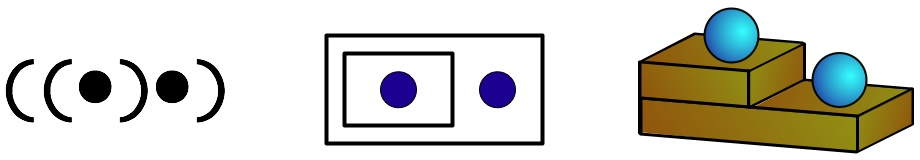The typographical representation of a planar container manifests as a matched pair of symbols. However the two “sides” of the parenthesis are aspects of a single boundary object, a single enclosure. Habitually we read the left parenthesis as “open” and the right as “close”, but the apparent linear sequence is incidental, not part of the intended meaning of the parens. Any interpretation that calls upon the ideas of a “left parenthesis” or a “right parenthesis” is in error. The only distinction a parens enclosure makes is that of inside and outside; the dual textual symbols are an artifact of typography. Typographical space is used to visually emphasize changes by deletion. In the image, units that remain vertically aligned are the same unit at different times during the transformation sequence.

## The Iconic Arithmetic Transformation Rules

The arithmetic of iconic numbers consists of putting together (addition and subtraction), substitution (multiplication and division), and Group and Merge (standardization). Grouping defines a notational shorthand, putting units into groups of the same size. The choice of how many units form a group is called the base of the system. Subtraction requires a second type of unit, the negative unit, together with a rule to Cancel opposite pairs of units. Here are the transformation rules of iconic arithmetic expressed in parens notation.

## Parens Notation for Dynamic Transformations

In the textual parens notation demonstrations that follow, each line describes a configuration of containers and units. The line directly below shows the application of a transformation rule. Both Merge and Cancel are deletion rules, the difference between the two sides of each equation is the presence or absence of structure. Deletions are particularly easy to read. For example, for the Merge rule, (a)(b)=(a b), the structure “)(” either goes away or is added. The application of deletion rules is indicated by the immediate absence (or presence) of structure on the next line down. Two non-deletion transformations, Group and Substitute, are highlighted for ease of reading. In each case the structure being changed is indicated by a different color. Here is how the transformations of iconic arithmetic are represented by before and after parens expressions.Ellipsis indicate any other content. The vertical stack of changes provides a sequential picture of the evolution of transformations applied to iconic forms. Parens has the advantage of spreading time out over the vertical spatial display, showing all changes “at the same time”. The cost is that the incidental sequential nature of parens notation both introduces artificial ordering operations and suppresses the natural parallelism of dynamic spatial interaction.

Merge, Unmerge and Cancel use typographical space to indicate their application. Substitution marked by a preceding asterisk indicates that a new form is to be inserted in place of the asterisk. That is, substitute a new form for the asterisk at the location of the asterisk in the existing configuration. A highlighted asterisk is a unit that has been substituted for a particular form. That is, substitute asterisk for a given form in the existing expression. In all cases, the asterisk is simply a unit that has been brought to our attention. Finally, note that the new parens formed by Group align directly underneath the two units being grouped (in base-2 only). Similarly, for Ungroup, the two new units align directly underneath the parens being deconstructed.

This page now shows the parens notation expression of the animations on the Container Numbers page and on the Binary Calculator page.

## Animations on the Container Numbers Page

The following parens transformation sequences replicate the container arithmetic animations shown on the Container Numbers page.

## Counting from 0 to 16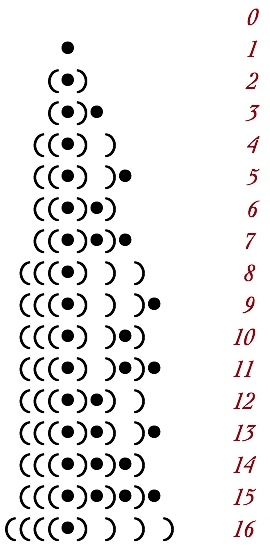### 7 + 5 = 12 and (–7) + (–5) = –12

Operations on positive and negative units are identical.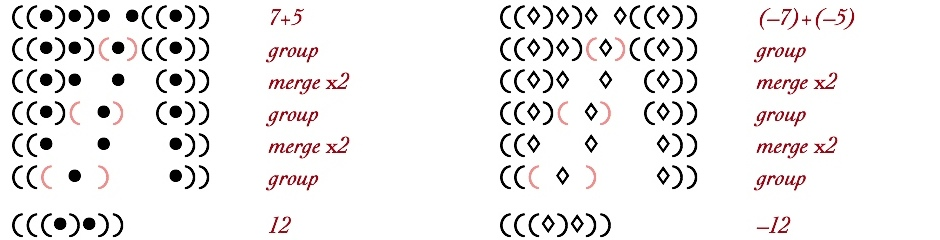### 2 + (–1) = 1 and 1 + (–2) = –1

Canceling occurs when opposite units share the same level of nesting. Both Ungroup and Unmerge are motivated by moving opposite units to the same level of nesting.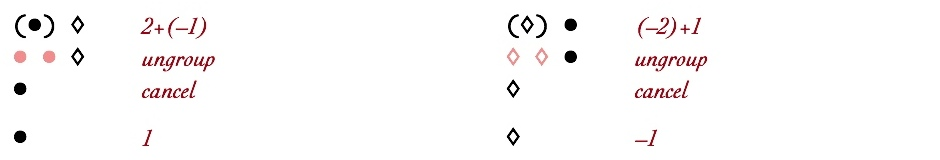### 2 + (–2) = 0 and 2 ÷ 2 = 1

The additive identity,0, and the multiplicative identity,1, are natural products of putting together and substituting.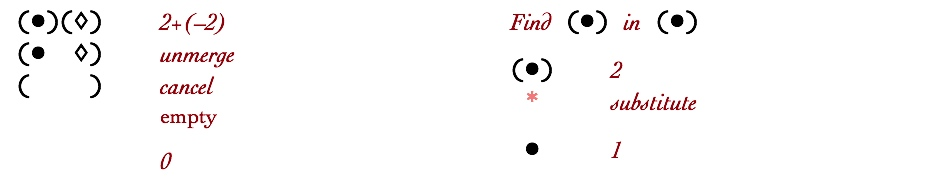### 7 + (–5) = 2 and (–7) + 5 = –2

Simplification follows an identical sequence regardless of which numbers are positive or negative.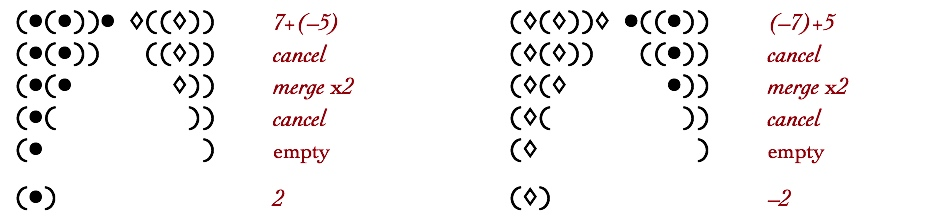### 3 x 1 = 3 and 1 x 3 = 3

The number of substitutions depends on what number is substituted into.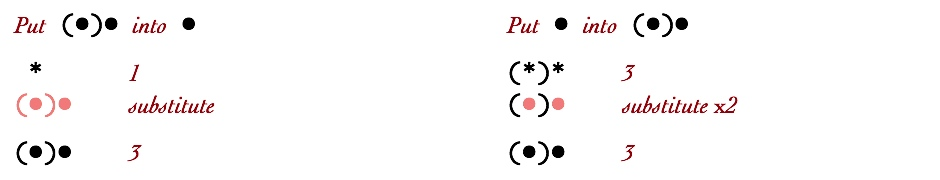### 2 x 3 = 6 and 3 x 2 = 6

The order of multiplication effects the amount of standardization effort but not the result.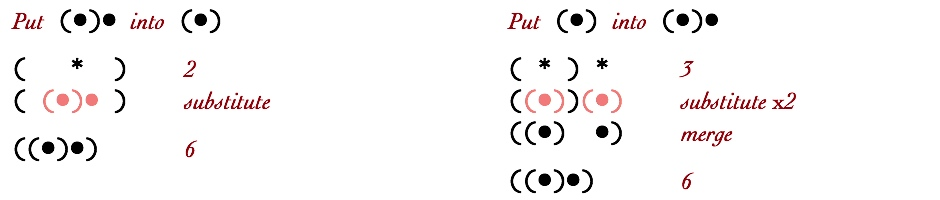### 5 x 7 = 35 and 7 x 5 = 35

Almost immediately after substitution, multiplied forms converge to the same standardization sequence. Here substitution followed by one Merge operation converts both orderings of multiplication to the same form.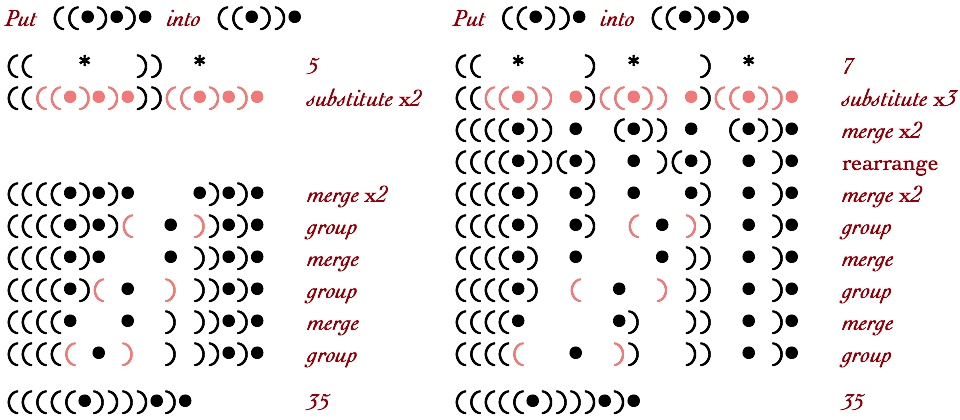### 35 ÷ 5 = 7 and 35 ÷ 7 = 5

In division, Unmerge and Ungroup are driven by finding the pattern of the divisor. The search rapidly diverges into different substitution sequences. In both cases the pattern of the divisor is identified by ungrouping the innermost units.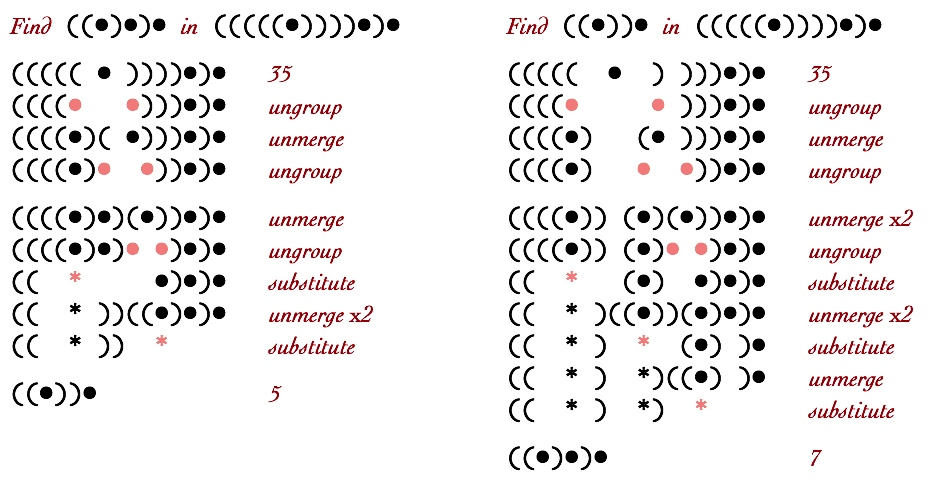## Animations on the Binary Calculator Page

The following parens transformation sequences replicate the container arithmetic animations shown on the Binary Calculator page. The Iconic Arithmetic Calculator implements more parallelism than shown on the Container Numbers page, but it does not implement all available parallelism. It was a design decision to limit parallelism to similar operations so that the Calculator animations would be easier to follow. The parens displays below use two notations for computational parallelism. Transformations are marked with a multiplier (such as merge x2) to indicate separate applications of the same rule on one line. As well, some transformations are marked with a token to indicate that in the animation that transformation occurs in parallel with other previously indicated transformations.

### Use of the Calculator, 435+(–65)+(–165)=225

This example shows all of the iconic arithmetic transformations working in concert.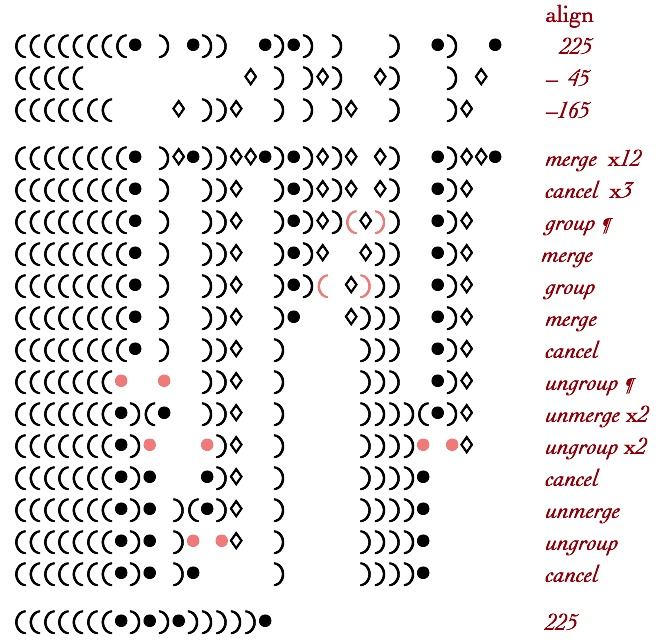### The Three Iconic Arithmetic Rules

Here is what each of the iconic arithmetic rules look like as an isolated parens sequence.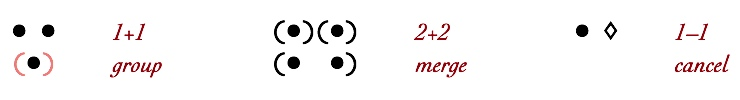### 1+1+1+1=4

An alternation of Group and Merge converts a unit-ensemble 4 into a depth-value 4.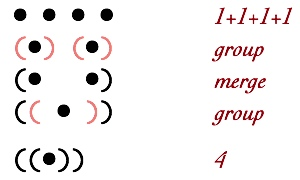### 2+2+2+2+2+2+2+2=16

Addition is an alternating sequence of Group and Merge. The first Merge x7 operation achieves boundary addition. The remaining transformation contribute to the standardization process.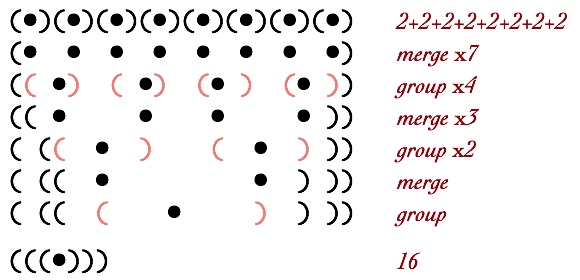### 8+8=16

It is an artifact of both the linear parens notation and the planar container notation that merging across different depths appears to involve multiple steps. Here three levels are merged concurrently. A three dimensional representation such as stacks of blocks is needed to show that merging across different levels is indeed parallel rather than sequential.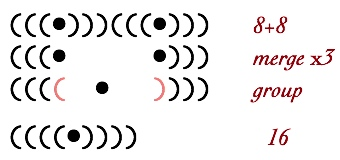### 5+7=12

This sum is shown above in the context of container numbers. It is also shown as an animation on the Network Numbers page.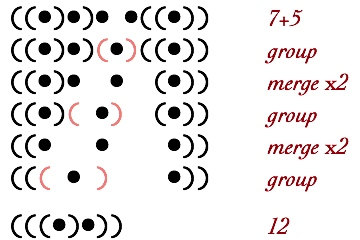### 1+31=32

This sum illustrates unavoidable sequential computation in addition. Strong parallelism means that each level can be processed independently. It also means that transfer of units across levels occurs sequentially.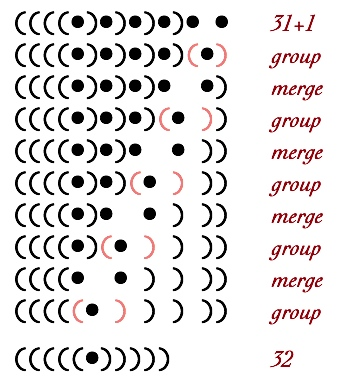### 545+154+324=1023

In this example Merge is all that is necessary.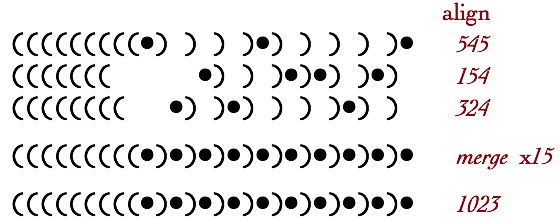### 51+348+110=509

Addition is achieved by the first parallel Merge. This results in a Group transformation at every level. These occur in parallel since levels are independent. Each of the resulting Merges finds the next lower level to be empty, thus completing the standardization process.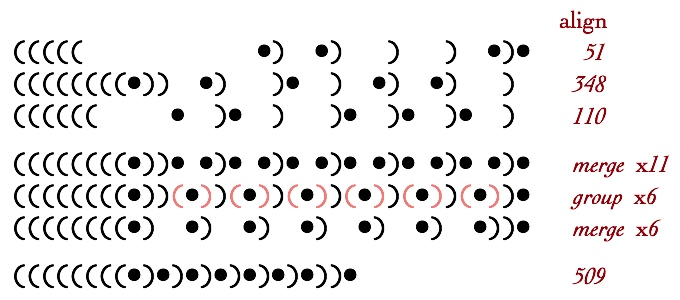### 1+2+3+4+5+6+7+8=36

Adding base-10 digits shows remarkable pattern structure in base-2 merging.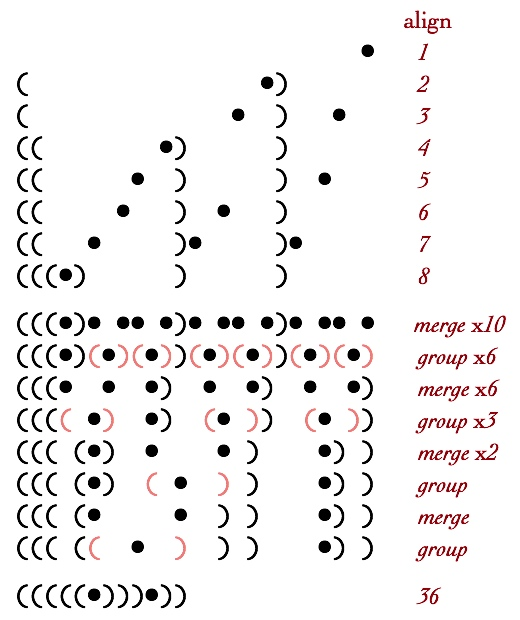### 15+15+15+15+15+15+15+15=120

This example shows logarithmic simplification. Sixteen groups convert to eight and then to four.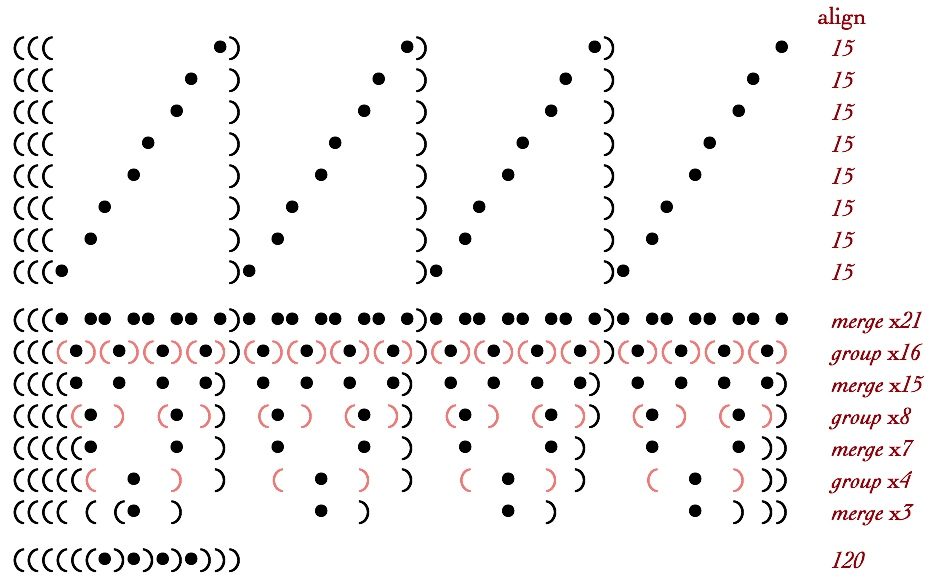### 1618+261+4401=6280

Although the base-10 numbers appear to share little structure, in base-2 their shared structure shows up as three nesting of the same pattern “))•)•)••“.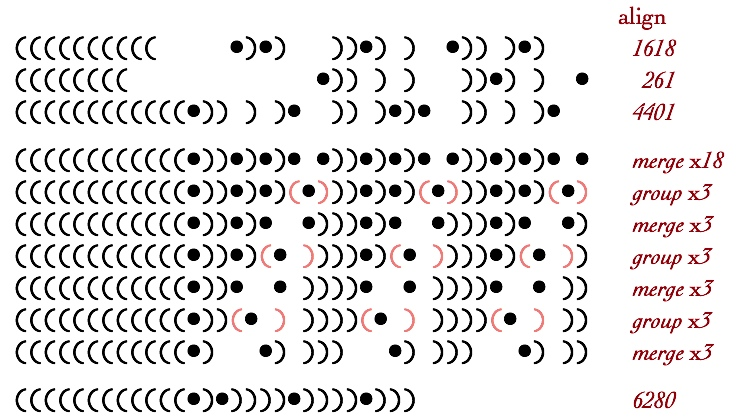### 2–1=1 and 1–2=–1

Subtraction introduces the necessity to Ungroup.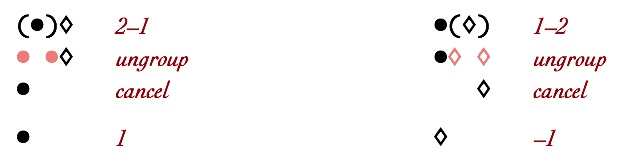### 32–1=31

This is the subtraction analog of the highly sequential 31+1 addition above.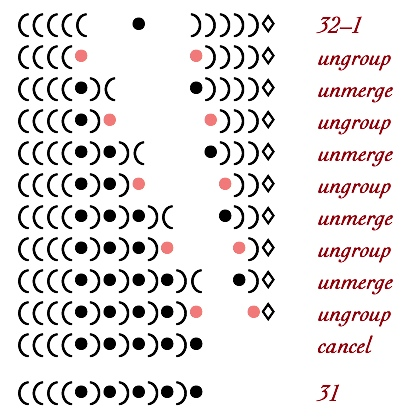### 215–87=128

In base-2, the opposite units align to immediately cancel the entirety of the negative number.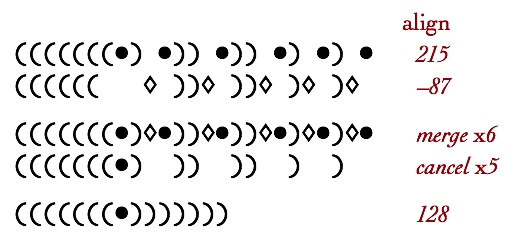### 235–225=10

This Cancel example shows the development of empty containers. Since nothing is interpreted as zero, an empty container would be transcribed conventionally as 2×0=0. An empty iconic container could be defined by the rule ( ) = . An alternative expression of this idea could be to create a corollary for the cancel rule: (•◊) = . The perspective adopted here is that, within the iconic arithmetic of numbers, empty containers are not defined, and are thus void-equivalent by default. Therefore the empty notation is the parens sequence is not a rule application, but is a reminder that empty parens are not valid forms.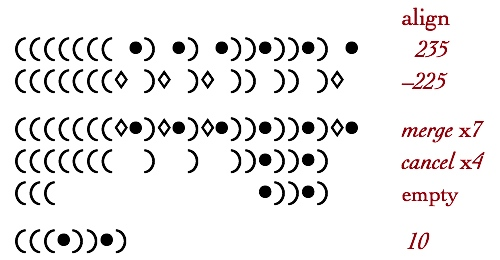### 42–213=–171

This example incorporates three parallel applications of Unmerge/Ungroup, in order to migrate units (here, negative units) to shallower levels. Again there is no global coordination, the Unmerge/Ungroup sequence is triggered by immediately adjacent units of opposite polarity.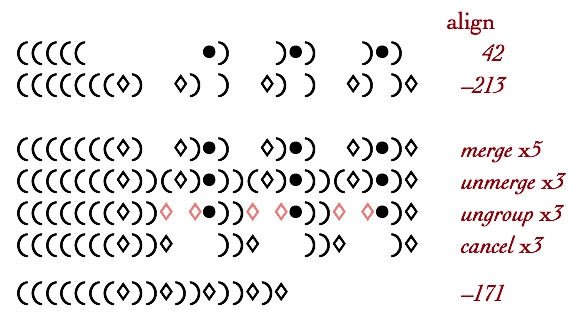### 9–4+5–3–8–7+1+9=2

A design decision for the Calculator implementation is to implement all Group operations prior to ungrouping to resolve mixed polarity forms. In this case, no ungrouping is necessary.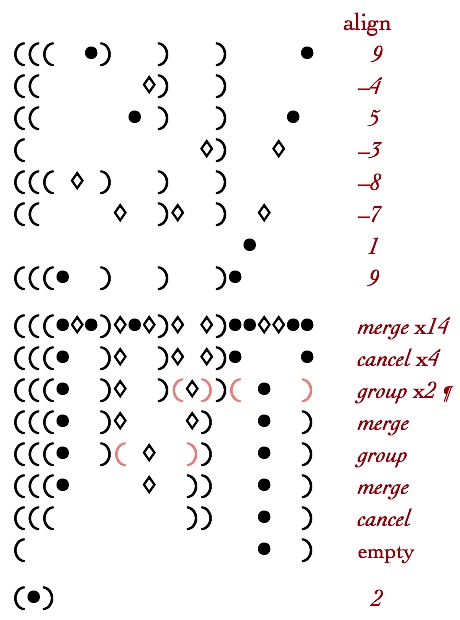### 9–4+5–3–8–5+1+9=4

This example is very similar to the prior example, with only one number changed. That change removes one of the group operations, leading to the necessity to Ungroup deeper units while standardizing the mixed polarity number.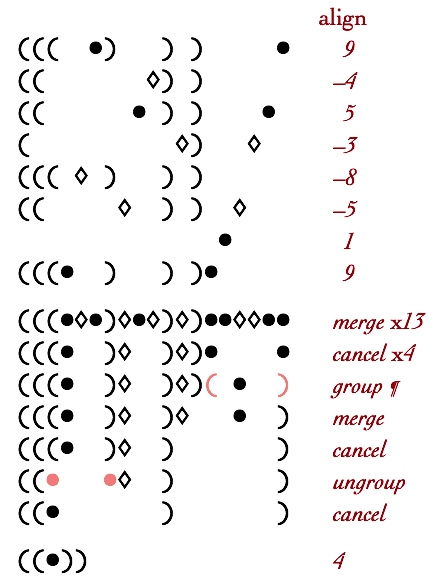### 9–1+9–7–6–6–6+9=1

Here is another example for which grouping resolves mixed levels. This example also shows the emergence of empty containers.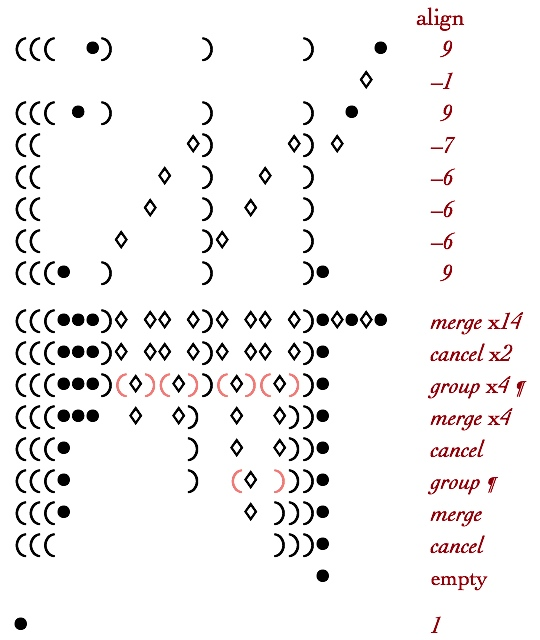### 200–20–90–90=0

After outer grouping in the first few steps of this example, inner ungrouping becomes necessary. At the last step, the result has no remaining units and is thus zero. The last Ungroup of the positive unit toward the bottom of the sequence could have been avoided by continuing to merge negative units inwards. This is an example of competition of parallel operations. The result, of course, is never effected. The precise type and sequence of reductive transformations, however, is a choice.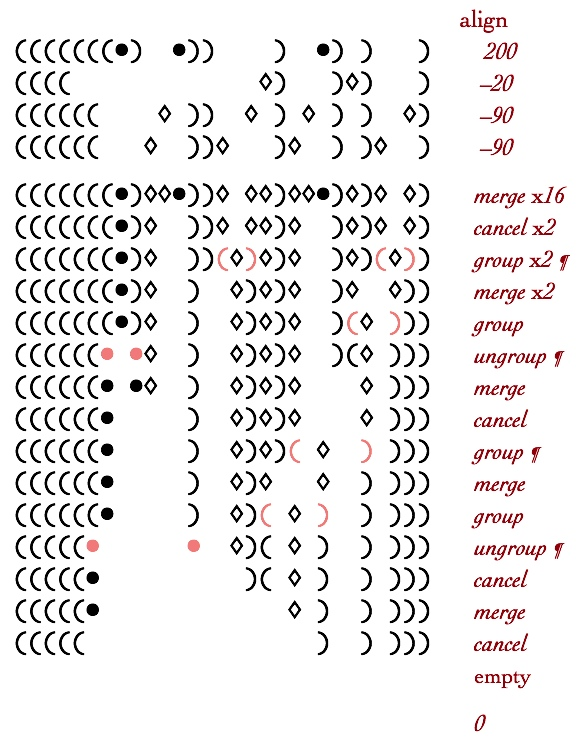### 234–45+329–171–164+10=193

This more complicated addition, by conventional standards at least, shows the same sequence of evolution as simpler mixed additions. After significant canceling the remaining units, regardless of polarity, group within each level. There is a subtle choice at step three, marked by the token. The two positive units could have grouped to Cancel the next deeper negative unit. However, due to the outer neighbor level also containing negative units, this grouping was suppressed.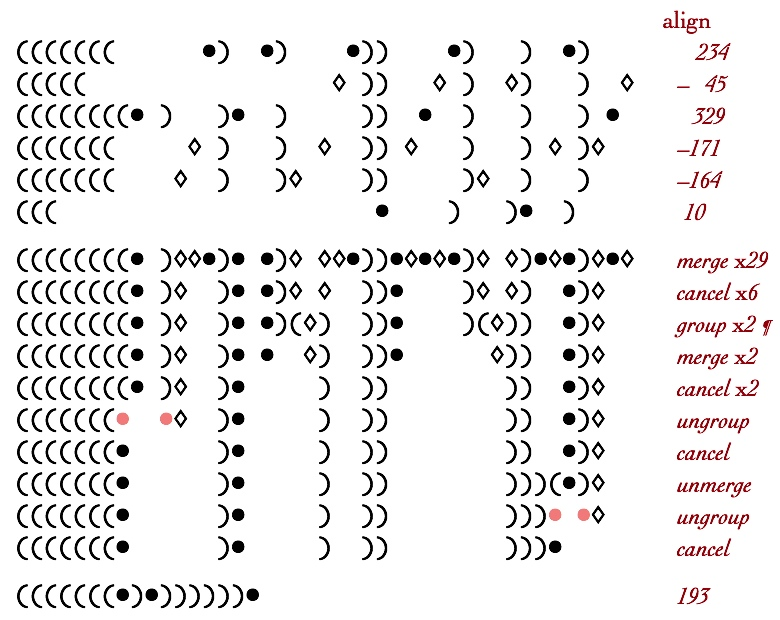### 211027–49037+48321–209808+90787=91290

Large numbers added together generate more nested levels, however due to parallelism within levels, the number of steps necessary to standardize the result grows slowly.